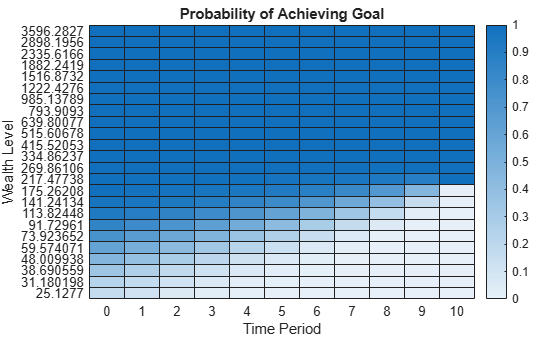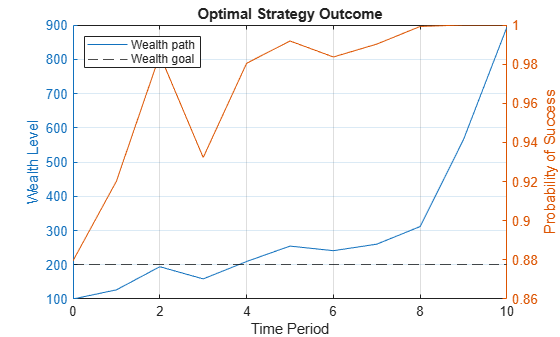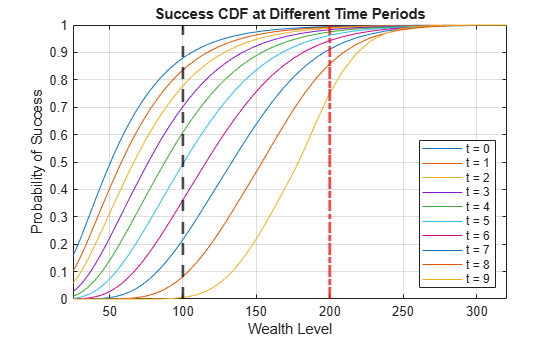# Dynamic Portfolio Allocation in Goal-Based Wealth Management for Multiple Time Periods

This example shows a dynamic programming strategy to maximize the probability of obtaining an investor's wealth goal at the end of the investment horizon. This dynamic programming strategy is known in the literature as a goal-based wealth management (GBWM) strategy. In GBWM, risk is not necessarily measured using the standard deviation, the value-at-risk, or any other common risk metric. Instead, risk is understood as the likelihood of not attaining an investor's goal. This alternative concept of risk implies that, sometimes, in order to increase the probability of attaining an investor’s goal, the optimal portfolio’s traditional risk (that is, standard deviation) must increase if the portfolio is underfunded. In other words, for the investor’s view of risk to decrease, the traditional view of risk must increase if the portfolio’s wealth is too low.

The objective of the investment strategy is to determine a dynamic portfolio allocation such that the portfolios chosen at each rebalancing period are on the traditional mean-variance efficient frontier and these portfolios maximize the probability of achieving the wealth goal $\mathit{G}$ at the time horizon $\mathit{T}$. This objective is represented as

$\underset{\left\{\mathit{A}\left(0\right),\mathit{A}\left(1\right),\dots ,\mathit{A}\left(\mathit{T}-1\right)\right\}}{\mathrm{max}}\text{\hspace{0.17em}\hspace{0.17em}}\mathbb{P}\left(\mathit{W}\left(\mathit{T}\right)\ge \mathit{G}\right)$,

where $\mathit{W}\left(\mathit{T}\right)$ is the terminal portfolio wealth and $\mathit{A}\left(\mathit{t}\right)$ are the possible actions and allocations at time $\mathit{t}=0,1,\dots ,\mathit{T}-1$.

The dynamic portfolio problem consists of the following parts:

• Time periods — Portfolio rebalancing periods

• Actions — Portfolio weights rebalancing

• States — Wealth levels

• Value function — Probability of achieving the goal at the end of the investment period

• Policy or strategy — Optimal actions at each state and time period

This example follows the GBWM strategy of Das . For an example of GBWM for a single time period, see the Single Period Goal-Based Wealth Management example.

### Inputs for Investment Wealth Goals

Specify the initial wealth.

`W0 = 100;`

Specify the target wealth at the end of the investment horizon.

`G = 200;`

Define the time horizon.

`T = 10;`

`load BlueChipStockMoments.mat`

### Rebalancing Period Actions for Efficient Portfolios

The action at each rebalancing period is to choose the weights of the investment portfolio for the next time period. In this example assume that the possible portfolio weights are associated with the portfolios on the efficient frontier. This example also assumes that the choice of possible portfolios is fixed throughout the full investment period. However, weights are not required to be on the efficient frontier. The only requirement is that the set of actions (portfolio choices) remains fixed throughout the investment period.

To simplify the computations, define a finite subset of the efficient portfolios for possible actions. Start by creating a `Portfolio` object with the asset information from `BlueChipStockMoments.mat`.

```p = Portfolio(AssetList=AssetList,AssetMean=AssetMean,... AssetCovar=AssetCovar);```

Annualize the asset returns and the variance-covariance matrix.

```p.AssetMean = 12*p.AssetMean; p.AssetCovar = 12*p.AssetCovar;```

Use `setBounds` to add constraints to the portfolio problem. Here, assets do not represent more than 10% of the portfolio wealth and shorting is not allowed.

`p = setBounds(p,0,0.1);`

Use `setBudget` to specify that the portfolio must be fully invested.

`p = setBudget(p,1,1);`

Compute the weights of `15` portfolios on the efficient frontier using `estimateFrontier`. These portfolios represent the possible actions at each rebalancing period.

```nPortfolios = 15; pwgt = estimateFrontier(p,nPortfolios);```

Plot the efficient frontier using `plotFrontier`.

`[prsk,pret] = plotFrontier(p,pwgt);`### States for Wealth Nodes

The states are defined as the wealth values at time $\mathit{t}$. To simplify the problem, discretize the wealth values to generate a wealth grid, ${\mathit{W}}_{\mathit{i}}$, for $\mathit{i}\in \left\{1,\dots ,{\mathit{i}}_{\mathrm{max}}\right\}$.${\mathit{W}}_{1}$ corresponds to the smallest possible wealth ${\mathit{W}}_{\mathrm{min}}$ and ${\mathit{W}}_{{\mathit{i}}_{\mathrm{max}}}$ corresponds to the largest possible wealth ${\mathit{W}}_{\mathrm{max}}$. To determine ${\mathit{W}}_{\mathrm{min}}$ and ${\mathit{W}}_{\mathrm{max}}$, assume that the evolution of wealth follows a geometric Brownian motion

$\mathit{W}\left(\mathit{t}\right)=\mathit{W}\left(0\right){\mathit{e}}^{\left(\mu -{\sigma \text{\hspace{0.17em}}}^{2}/2\right)\mathit{t}+\sigma \sqrt{\mathit{t}}\mathit{Z}}$,

where $\mathit{Z}$ is a standard normal random variable. GBWM does not require that the wealth evolution follow a Brownian motion. However, the technique in this example requires the evolution model to be Markovian. Therefore, this example assumes that the probability of being at a certain state at time $\mathit{t}+1$ only depends on the state at time $\mathit{t}$ and the action taken.

This example also assumes that $\mathit{Z}$ realistically takes values between $-3$ and $3$. When $\mathit{Z}=-3$, ${\mathit{W}}_{\mathrm{min}}$ corresponds to the smallest value obtained in the trajectory of the Brownian motion setting, $\mu$ is the smallest possible portfolio return ${\mu }_{\mathrm{min}}$, and $\sigma$ is the largest possible portfolio risk ${\sigma }_{\mathrm{max}}$. Likewise, when $\mathit{Z}=3$, ${\mathit{W}}_{\mathrm{max}}$ corresponds to the largest value in the trajectory, $\mu$ is the largest possible portfolio return ${\mu }_{\mathrm{max}}$, and $\sigma$ is the largest possible portfolio risk ${\sigma }_{\mathrm{max}}$. For ${\mathit{W}}_{\mathrm{max}}$, use ${\sigma }_{\mathrm{max}}$ because the term in the exponential is increasing for all $\sigma \le 3/\sqrt{\mathit{t}}$; if ${\sigma }_{\mathrm{max}}>3/\sqrt{\mathit{t}}$, use $\sigma =3/\sqrt{\mathit{t}}$ instead.

Define ${\mu }_{\mathrm{min}}$, ${\mu }_{\mathrm{max}}$, ${\sigma }_{\mathrm{min}}$, and ${\sigma }_{\mathrm{max}}$.

```% Define return limits. mu_min = pret(1); mu_max = pret(end); % Define volatility limits. sigma_min = prsk(1); sigma_max = prsk(end);```

The smallest realistic value of $\mathit{W}$ is

${\stackrel{ˆ}{\mathit{W}}}_{\mathrm{min}}=\underset{\tau \in \left\{0,1,\dots ,\mathit{T}\right\}}{\mathrm{min}}\text{\hspace{0.17em}}\left\{\mathit{W}\left(0\right){\mathit{e}}^{\left({\mu }_{\mathrm{min}}-\frac{{\sigma }_{\mathrm{max}}^{2}}{2}\right)\tau -3{\sigma }_{\mathrm{max}}\sqrt{\tau }}\right\}$.

```% Define the minimum possible wealth. timeVec = 0:T; WMinVec = W0*exp((mu_min-(sigma_max^2)/2)*timeVec- ... 3*sigma_max*sqrt(timeVec)); WHatMin = min(WMinVec);```

The largest realistic value of $\mathit{W}$ is

${\stackrel{ˆ}{\mathit{W}}}_{\mathrm{max}}=\underset{\tau \in \left\{0,1,\dots ,\mathit{T}\right\}}{\mathrm{max}}\text{\hspace{0.17em}}\left\{\mathit{W}\left(0\right){\mathit{e}}^{\left({\mu }_{\mathrm{max}}-\frac{{\sigma }_{\mathrm{max}}^{2}}{2}\right)\tau +3{\sigma }_{\mathrm{max}}\sqrt{\tau }}\right\}$.

```% Define the maximum possible wealth. WMaxVec = W0*exp((mu_max-(sigma_max^2)/2)*timeVec+ ... 3*sigma_max*sqrt(timeVec)); WHatMax = max(WMaxVec);```

Now, you can fill in the grid points between ${\stackrel{ˆ}{\mathit{W}}}_{\mathrm{min}}$ and ${\stackrel{ˆ}{\mathit{W}}}_{\mathrm{max}}$. When you set $\mathit{Z}=1$and $\mathit{t}=1\text{\hspace{0.17em}}$and ignore the drift term $\left(\mu -{\sigma }^{2}/2\right)\mathit{t}$, note that $\sigma$ is proportional to the logarithm of the wealth. Thus, you can work in logarithmic space and add a grid point every ${\sigma }_{\mathrm{min}}/{\rho }_{\mathrm{grid}}$ units. ${\rho }_{\mathrm{grid}}$ is an exogenous parameter that determines the density of the grid. As ${\rho }_{\mathrm{grid}}$ grows, the number of wealth nodes increases.

```% Transform to the logarithmic space. lWHatMin = log(WHatMin); lWHatMax = log(WHatMax); % Define the grid in the logarithmic space. rho = 3; lWGrid = (lWHatMin:sigma_min/rho:lWHatMax)';```

To guarantee that there exists a point in the wealth grid that matches $\mathit{W}\left(0\right)$, shift the logarithmic grid downwards by the smallest shift such that one of those values matches the logarithm of the initial portfolio value.

```% Compute the shift for wealth grid. lW0 = log(W0); difference = lWGrid-lW0; minDownShift = min(difference(difference>=0)); % Define the wealth nodes. WGrid = exp(lWGrid - minDownShift); nWealthNodes = size(WGrid,1); % Number of wealth nodes```

### Value Function and Optimal Strategy

The problem in this example is a multi-period optimization problem. The goal is to find the action at each rebalancing period that maximizes the probability that the wealth exceeds the target goal at the end of the investment period. To solve this problem, use the Bellman equation, which in this case is given by

$V\left({W}_{i}\left(t\right)\right)=\underset{\pi }{\mathrm{max}}\left[{\sum }_{j}^{}V\left({W}_{j}\left(t+1\right)\right)p\left({W}_{j}\left(t+1\right)|{W}_{i}\left(t\right),\pi \right)\right]$.

The value function $\mathit{V}\left({\mathit{W}}_{\mathit{i}}\left(\mathit{t}\right)\right)$ represents the probability of exceeding the wealth goal $\mathit{G}$ at time $\mathit{T}$, given that at time $\mathit{t}$, the wealth level is ${\mathit{W}}_{\mathit{i}}$. Simultaneously, $\mathit{p}\left({\mathit{W}}_{\mathit{j}}\left(\mathit{t}+1\right)|{\mathit{W}}_{\mathit{i}}\left(\mathit{t}\right),\pi \right)$ represents the probability of moving to wealth node $\mathit{j}$ at time $\mathit{t}+1$, given that at time $\mathit{t}$, you are at wealth node $\mathit{i}$ and you chose portfolio $\pi$ as the rebalancing action.

### Compute Transition Probabilities

Since this example assumes that the wealth goal follows a geometric Brownian motion, the transition probabilities of being in wealth node ${\mathit{W}}_{\mathit{j}}$ at time $\mathit{t}+1$, given that you are at wealth node ${\mathit{W}}_{\mathit{i}}$ at time $\mathit{t}$, are time-homogeneous. Thus, the transition probability for a given portfolio choice $\pi$ is defined as

$\mathit{p}\left({\mathit{W}}_{\mathit{j}}\left(\mathit{t}+1\right)|{\mathit{W}}_{\mathit{i}}\left(\mathit{t}\right),\pi \right)=\mathbb{P}\left({\mathit{W}}_{\mathit{j}}\le \mathit{W}\left(\mathit{t}+1\right)<{\mathit{W}}_{\mathit{j}+1}|{\mathit{W}\left(\mathit{t}\right)=\mathit{W}}_{\mathit{i}},\pi \right)=\stackrel{\sim }{\mathit{p}}\left({\mathit{W}}_{\mathit{j}+1}|{\mathit{W}}_{\mathit{i}},\pi \right)-\stackrel{\sim }{\mathit{p}}\left({\mathit{W}}_{\mathit{j}}|{\mathit{W}}_{\mathit{i}},\pi \right),\text{\hspace{0.17em}\hspace{0.17em}}\forall \text{\hspace{0.17em}}\mathit{t}\in \left\{0,\dots ,\mathit{T}\right\}$,

where

$\stackrel{\sim }{\mathit{p}}\left({\mathit{W}}_{\mathit{k}}|{\mathit{W}}_{\mathit{i}},\pi \right)=\Phi \left(\frac{1}{{\sigma }_{\pi }}\left(\mathrm{ln}\left(\frac{{\mathit{W}}_{\mathit{k}}}{{\mathit{W}}_{\mathit{i}}}\right)-\left({\mu }_{\pi }-\frac{{{\sigma }_{\pi }}^{2}}{2}\right)\right)\right)$,

$\Phi$ is the cumulative distribution function of the standard normal random variable, and ${\mu }_{\pi }$ and ${\sigma }_{\pi }$ are the return and risk of portfolio $\pi$. If $\mathit{j}=1$,

$\mathit{p}\left({\mathit{W}}_{1}\left(\mathit{t}+1\right)|{\mathit{W}}_{\mathit{i}}\left(\mathit{t}\right),\pi \right)=\stackrel{\sim }{\mathit{p}}\left({\mathit{W}}_{2}|{\mathit{W}}_{\mathit{i}},\pi \right),\text{\hspace{0.17em}\hspace{0.17em}}\forall \text{\hspace{0.17em}}\mathit{t}\in \left\{0,\dots ,\mathit{T}\right\}$,

and if $\mathit{j}={\mathit{i}}_{\mathrm{max}}$,

$\mathit{p}\left({\mathit{W}}_{{\mathit{i}}_{\mathrm{max}}}\left(\mathit{t}+1\right)|{\mathit{W}}_{\mathit{i}}\left(\mathit{t}\right),\pi \right)=1-\stackrel{\sim }{\mathit{p}}\left({\mathit{W}}_{{\mathit{i}}_{\mathrm{max}}}|{\mathit{W}}_{\mathit{i}},\pi \right),\text{\hspace{0.17em}\hspace{0.17em}}\forall \text{\hspace{0.17em}}\mathit{t}\in \left\{0,\dots ,\mathit{T}\right\}$.

Note that this example computes transition probabilities differently from the transition probabilities as described by Das , to keep a consistent mapping between $\mathit{W}\left(\mathit{t}\right)$ and a unique wealth node index.

```% Compute the transition probabilities for different portfolios: % pTransition(i,j,k) = p(Wj|Wi,mu_k) pTransition = zeros(nWealthNodes,nWealthNodes,nPortfolios); for k = 1:nPortfolios % Get the return and variance of portfolio k. mu = pret(k); sigma = prsk(k); % Compute p tilde before the cdf. pTilde = 1/sigma*(log(WGrid'./WGrid) - (mu-(sigma^2)/2)); % Auxiliary matrices for edge cases (j = 1 and j = i_max) pTildeAuxUB = [pTilde(:,2:end) inf(nWealthNodes,1)]; pTildeAuxLB = [-inf(nWealthNodes,1) pTilde(:,2:end)]; % Compute the transition probabilities for portfolio k. pTransition(:,:,k) = normcdf(pTildeAuxUB)-normcdf(pTildeAuxLB); end```

### Maximize Probability of Achieving Investor's Goal

Since the value function is the probability of achieving the wealth investment goal $\mathit{G}$ at the final time step, you know that the value function at $\mathit{t}=\mathit{T}$ is given by

`$\mathit{V}\left({\mathit{W}}_{\mathit{i}}\left(\mathit{t}\right)\right)=\left\{\begin{array}{ll}0& \mathrm{if}\text{\hspace{0.17em}}{\mathit{W}}_{\mathit{i}}\left(\mathit{T}\right)<\mathit{G}\\ 1& \mathrm{if}\text{\hspace{0.17em}}{\mathit{W}}_{\mathit{i}}\left(\mathit{T}\right)\ge \mathit{G}\end{array}$`

```% Intialize the matrix that stores the value function at different % wealth nodes and time periods. V = zeros(nWealthNodes,T+1); % Define the Value function at t = T. V(:,end) = (WGrid >= G);```

To compute the value of $\mathit{V}\left({\mathit{W}}_{\mathit{i}}\left(\mathit{t}\right)\right)$ for $\mathit{t}<\mathit{T}$, you need to go backwards in time. You start at $\mathit{t}=\mathit{T}-1$ to determine the portfolio that achieves the maximum in the Bellman equation and $\mathit{V}\left({\mathit{W}}_{\mathit{i}}\left(\mathit{T}-1\right)\right)$. Then continue with $\mathit{t}=\mathit{T}-2$, then $\mathit{t}=\mathit{T}-3$, until you reach $\mathit{t}=0$. The value of $\mathit{V}\left(\mathit{W}\left(0\right)\right)$ is the optimal probability of attaining the wealth goal $\mathit{G}$ from the initial wealth $\mathit{W}\left(0\right)$.

```% Compute the optimal value function and portfolio at different % wealth nodes and time periods. portIdx = zeros(nWealthNodes,T); for t = T:-1:1 pTilde = zeros(nWealthNodes,nPortfolios); for k = 1:nPortfolios % sum_j ( V(Wj(t+1)) * p(Wj|Wi,mu_k) ) pTilde(:,k) = pTransition(:,:,k)*V(:,t+1); end % Choose the portfolios that achieve the best probabilities. [V(:,t),portIdx(:,t)] = max(pTilde,[],2); end```

Note that `V(i,t)` is $\mathit{V}\left({\mathit{W}}_{\mathit{i}}\left(\mathit{t}-\mathrm{1}\right)\right)$, which denotes the optimal probability of achieving the wealth goal from wealth node ${\mathit{W}}_{\mathit{i}}$ at time period $\mathit{t}-1$. On the other hand, `portIdx(i,t)` defines the optimal portfolio to be chosen at time period $\mathit{t}-1$ if you are at wealth node ${\mathit{W}}_{\mathit{i}}$. The value of $\mathit{t}$ is shifted down for `V(i,t)` and `portIdx(i,t)`, given that array indices start at `1` and $\mathit{t}$ starts at `0`.

Use `heatmap` to plot the value function.

```% Plot a heatmap of the value function. figure; heatmap(0:T,flip(WGrid(1:5:end)),flip(V(1:5:end,:))) xlabel("Time Period") ylabel("Wealth Level") title("Probability of Achieving Goal")```The figure shows how the probability of achieving the goal becomes larger as the initial wealth grows. Also, this figure demonstrates that as time passes, the current wealth must be higher than in the previous periods to get the same probability of achieving the goal.

Use `heatmap` to plot the optimal portfolio.

```% Plot a heatmap of optimal portfolio. figure; heatmap(0:T-1,flip(WGrid(1:5:end)),flip(portIdx(1:5:end,:))) xlabel("Time Period") ylabel("Wealth Level") title("Portfolio Aggressiveness")```This figure shows that the higher the wealth, the lower the number of the optimal portfolio. Since the number of the portfolio is directly related to the aggressiveness of the strategy, you can see that the wealthier the portfolio, the less risk is needed to achieve the final wealth goal. When the portfolio has less money, then the optimal portfolio is the one with a larger expected return, even at the cost of increasing the volatility. Also, note that the closer the time period is to the end of the investment horizon, the choice of the optimal portfolio becomes polarized, where the portfolio is either extremely aggressive or extremely conservative.

### Implement Optimal Strategy

The following describes the workflow to implement the optimal strategy:

1. At $\mathit{t}=0$, choose the optimal strategy shown in `portIdx(i,j)` such that $\mathit{i}$ is the index associated with the wealth node that satisfies ${\mathit{W}}_{\mathit{i}}\le \mathit{W}\left(0\right)<{\mathit{W}}_{\mathit{i}+1}$ and $\mathit{j}=\mathit{t}+1$. Also, let ${\mathit{A}}^{*}\left(0\right)=$`portIdx(i,j).`

2. Rebalance the portfolio to match the weights in `pwgt(:,`${\mathit{A}}^{*}\left(0\right)$`)`. Then, define ${\pi }^{*}\left(0\right)=$`pwgt(:,`${\mathit{A}}^{*}\left(0\right)$`). `

3. Either using historical data for time period $\mathit{t}=0$ or simulating one step of a Brownian motion, obtain $\mathit{W}\left(1\right)$, given that you started at $\mathit{W}\left(0\right)$, and chose portfolio ${\pi }^{*}\left(0\right)$.

4. Repeat steps 1 through 3 for $\mathit{t}=1,\dots ,\mathit{T}-1$.

```% Simulate one path following the optimal strategy. rng('default') [Z,WPath,portPath,VPath] = DynamicPortSim(W0,WGrid,pret,prsk, ... portIdx,V);```

Present a table with the results of the simulation.

```simulation = table((0:T)',Z,WPath,portPath,VPath,'VariableNames', ... {'t','Z','Wealth','OptPort','ProbOfSuccess'})```
```simulation=11×5 table t Z Wealth OptPort ProbOfSuccess __ ________ ______ _______ _____________ 0 0.53767 100 12 0.87953 1 1.8339 126.69 11 0.92018 2 -2.2588 194.27 11 0.98474 3 0.86217 158.68 11 0.9323 4 0.31877 209.52 11 0.98044 5 -1.3077 254.46 10 0.99184 6 -0.43359 241.04 10 0.98366 7 0.34262 260.14 8 0.99024 8 3.5784 311.62 5 0.99927 9 2.7694 566.28 1 1 10 NaN 894.5 NaN 1 ```

Note that as the probability of attaining the wealth goal (the value function) increases, the aggressiveness (number) of the optimal portfolio decreases. Now, you can plot the path of the wealth against the probability of achieving the wealth goal at the end of the investment period.

```figure; % Plot the wealth path. yyaxis left plot(0:T,WPath) hold on yline(G,'--k'); ylabel("Wealth Level"); % Plot the value function path. yyaxis right plot(0:T,VPath) ylabel("Probability of Success"); % Specify the title, labels, and legends for the plot. title("Optimal Strategy Outcome") xlabel("Time Period"); legend("Wealth path","Wealth goal",Location="nw"); grid on hold off```At $\mathit{t}=4$, the wealth goal has been achieved. However, because of the uncertainty in the problem, the probability of success is not yet 1. Furthermore, trying different paths (random seeds) shows how even a monotonic increase in the wealth does not translate to a monotonic increase in the probability of success. This behavior occurs because the wealth increase at later time periods should be higher than at earlier time periods to have the same increase in the probability of success.

Plot the cumulative distribution function (CDF) of attaining the goal wealth at different time periods.

```% Plot the cumulative distribution function (CDF) of success at % different time periods. figure nWN = 60; plot(WGrid(1:nWN),V(1:nWN,1:end-1)) xline(W0,'--k','LineWidth',2); % Initial wealth xline(G,'-.r','LineWidth',2); % Goal wealth xlim(WGrid([1,nWN])) % Specify the title, labels, and legends. title('Success CDF at Different Time Periods') xlabel('Wealth Level') ylabel('Probability of Success') legend("t = "+num2str((0:T-1)'),Location="se") grid on```This figure shows how as the time period approaches the investment horizon, the CDF shifts to the right and becomes steeper. This shift demonstrates that changes in the wealth level have a higher impact toward the end of the investment horizon. For example, an increase of 100 to 110 wealth points at $\mathit{t}=0$ only increases the probability of success by 3%, while the same wealth increase at $\mathit{t}=4$ increases the probability of success by 7%.

### Local Functions

```function [Z,WPath,portPath,VPath] = DynamicPortSim(W0,WGrid,... pret,prsk,portIdx,V) % Define time periods. T = size(V,2)-1; % Define standard normal random variables for the Brownian motion. Z = randn(T,1); Z = [Z;nan]; % No randomness at the final time period % Find the wealth node index of the initial wealth. tol = 1e-8; % Account for numerical inaccuracies difference = WGrid-W0; % Assign to wealth node j such that W_j <= W(0) < W_{j+1} WNodeIdx = find(difference<=tol,1,'last'); % Run the simulation. WPath = [W0; zeros(T,1)]; portPath = [portIdx(WNodeIdx,1); zeros(T,1)]; VPath = [V(WNodeIdx,1); zeros(T,1)]; for t = 1:T % Get the return and variance of optimal portfolio at time t. mu = pret(portIdx(WNodeIdx,t)); sigma = prsk(portIdx(WNodeIdx,t)); % Compute the wealth at time t+1 following the Browninan motion. WPath(t+1) = WPath(t)*exp(mu-(sigma^2)/2+sigma*Z(t)); % Compute the wealth node index corresponding to the current wealth. difference = WGrid-WPath(t+1); % Assign to wealth node j such that W_j <= W(t+1) < W_{j+1} WNodeIdx = find(difference <= tol,1,'last'); % Store the optimum strategy and value function. if t<T portPath(t+1) = portIdx(WNodeIdx,t+1); else portPath(t+1) = nan; end VPath(t+1) = V(WNodeIdx,t+1); end end```

### References

 Das, Sanjiv R., Daniel Ostrov, Anand Radhakrishnan, and Deep Srivastav. “Dynamic Portfolio Allocation in Goals-Based Wealth Management.” Computational Management Science 17, no. 4 (December 2020): 613–40. Available at: https://doi.org/10.1007/s10287-019-00351-7.